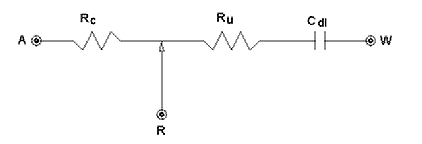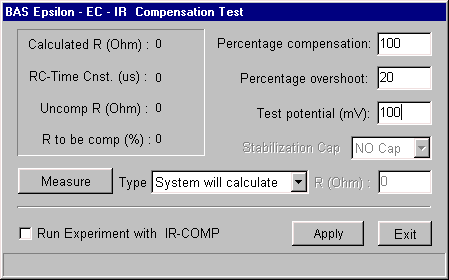# iR Compensation

In any potentiostatic experiment, it is assumed that the potential drop across the interfacial region at the working electrode is the same as the potential applied between the reference and working electrode. However, this is not true, since there is some iR drop between these two electrodes due to solution resistance. This resistance can be lowered by addition of supporting electrolyte, and in many cases does not need to be considered. However, there are instances where it is detrimental to the experiment; in these cases, it can be compensated for electronically. This is achieved on the epsilon using positive feedback iR compensation, which is set up using the iR Compensation Test dialog box, which is accessed using the IR-COMP button in Change Parameters dialog boxes.

One problem with positive feedback iR compensation is determining the amount of compensation to use, since too much feedback can drive the electronics into oscillation, which can have a deleterious effect on both the electronics and the electrodes. The automatic iR compensation option on the epsilon prevents this by first measuring the uncompensated solution resistance, followed by incremental compensation and circuit stability testing. These features are described below, together with a procedure for setting up iR compensation.

## Measurement of Uncompensated ResistanceFigure 1. Model of the electrochemical cell used for iR compensation.

In this measurement, the electrochemical cell is considered to be electronically equivalent to an RC circuit (Fig1); that is, the uncompensated resistance (Ru) is in series with the double-layer capacitance (Cdl). Since a faradaic impedance is not considered as part of this model, the Test potential must be at a value at which no faradaic process occurs. A potential step is applied at this potential and the current is sampled at set points after the step is applied. The current decays exponentially (Fig2), and the initial current (Io) is calculated by extrapolating back to zero time. Since E = IoRu, Ru can be calculated from this measurement. To reduce any error, this measurement is performed multiple times, and the results averaged.Figure 2. Exponential decay of the current response of the above RC series circuit following the applied potential step.

## Compensation and Circuit Stability Testing

Compensation is achieved by positive feedback into the potentiostat. However, problems due to circuit instability can arise, even when the degree of compensation is significantly less than 100%. Therefore, the amount of positive feedback is increased incrementally, and the stability of the circuit is tested after each increment. The amount of positive feedback is increased until the (user-specified) % value is obtained (default = 100%), or the system does not pass the stability test.Figure 3. Current response for the stability test showing the "ringing" effect.

In the stability test, the current following a potential step at the Test potential is again measured. As the percent compensation increases, the current response at first exhibits a "ringing effect" following exponential decay, which is a precursor to oscillation (Fig3). The degree of pre-oscillation ringing can quantitated by a term defined as overshoot, which the ratio of a minimum (net negative) current value (Imin) to a maximum current value (Imax) expressed as a percentage: that is, overshoot = (Imin/Imax) x 100. The maximum allowable Percentage overshoot is defined by the user (default = 20%). If the Percentage overshoot value measured by the stability test is less than the maximum allowable value, then compensation is increased. If it is greater, and the desired level of compensation has not yet been achieved, a capacitor is inserted between the reference and auxiliary electrodes to stabilize the circuit, and the testing is continued until the desired level of compensation is achieved or the Percentage overshoot value is exceeded (if this occurs, the amount of compensation to be used in the experiment is slightly decreased from this value). One way to increase the level of compensation is to increase the Percentage overshoot percentage. It is usually safe to go up to 40%.

If the desired compensation cannot be obtained using automatic iR Compensation, then a user-specified compensation resistance can be applied. If this option is used, then a stabilizing capacitor may also need to be selected manually.

## Setting up iR Compensation

iR compensation is set up using the IR Compensation Test dialog box which is accessed using the IR-COMP button in the Change Parameters dialog boxes.Figure 4. IR Compensation Test dialog box.

1. The simplest method for using iR compensation is to use the manual option (Type = User assign value). Enter the required resistance for the R (ohm), and make sure that the Run Experiment with IR-COMP check box is checked. A Stabilization Capacitor may be required for electronic stability (two are available - Large Cap and Small Cap). However, it is generally better to use the automatic option (Type = System will calculate), since using too high a resistance can have a deleterious effect on the electrode, the electronics, and the experimental data.
2. If the System will calculate (recommended) option is to be used, enter a value for the Test potential (mV) (note that this should be at a value at which there is no faradaic reaction). The size of the Stabilization Capacitor is selected by the software.
3. Click the Measure button to start the test. The following results are listed:
• The uncompensated resistance (Calculated R)
• The RC- time constant of the cell (RC-Time Cnst.)
• Uncompensated resistance remaining after compensation (Uncomp R)
• Percentage of the uncompensated resistance to be used in positive feedback (R to be comp.)
4. Once the iR Compensation Test has been completed, make sure that Run Experiment with IR-COMP box is checked, and then click Apply before exiting the dialog box.

Back to Table of Contents